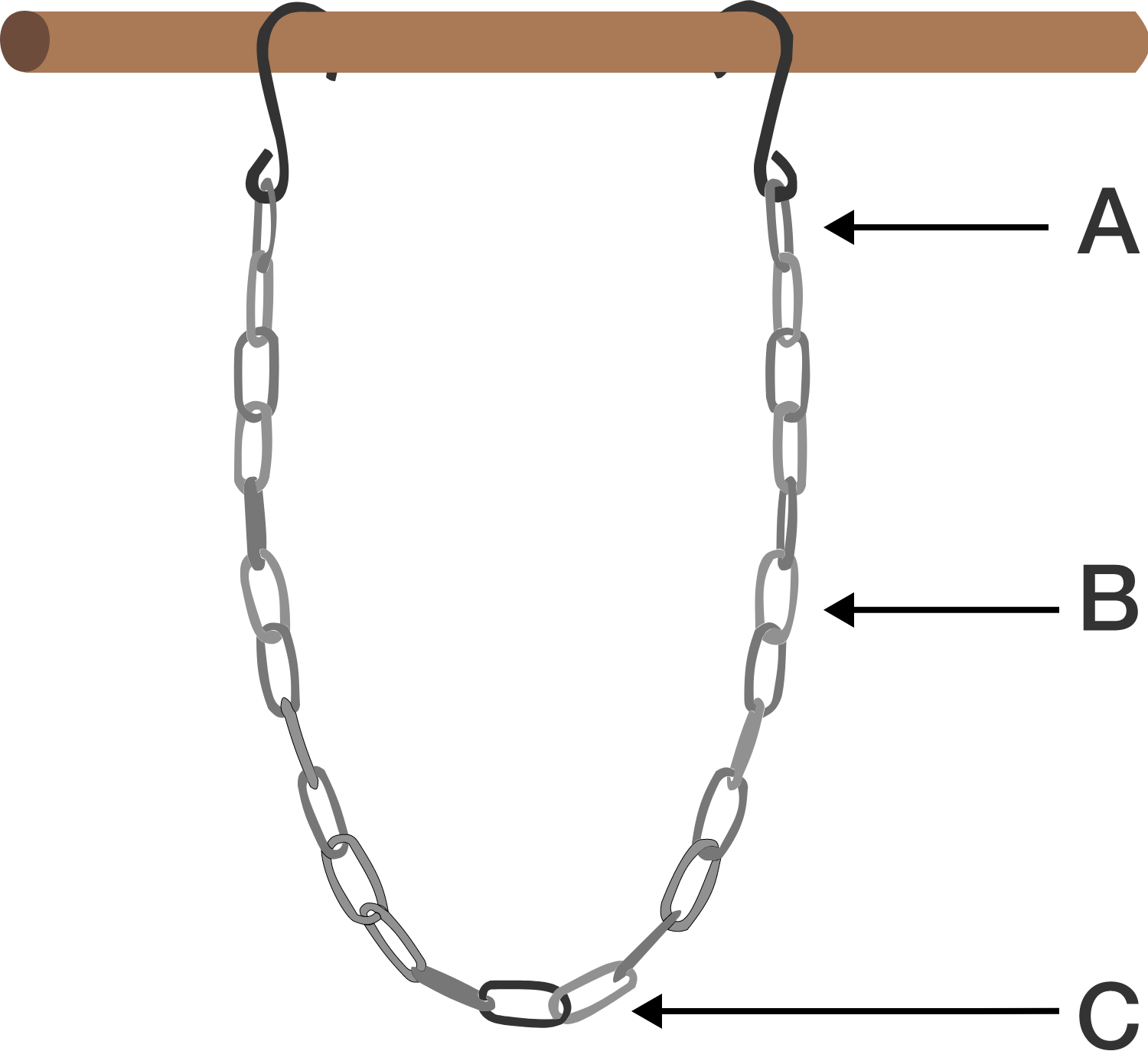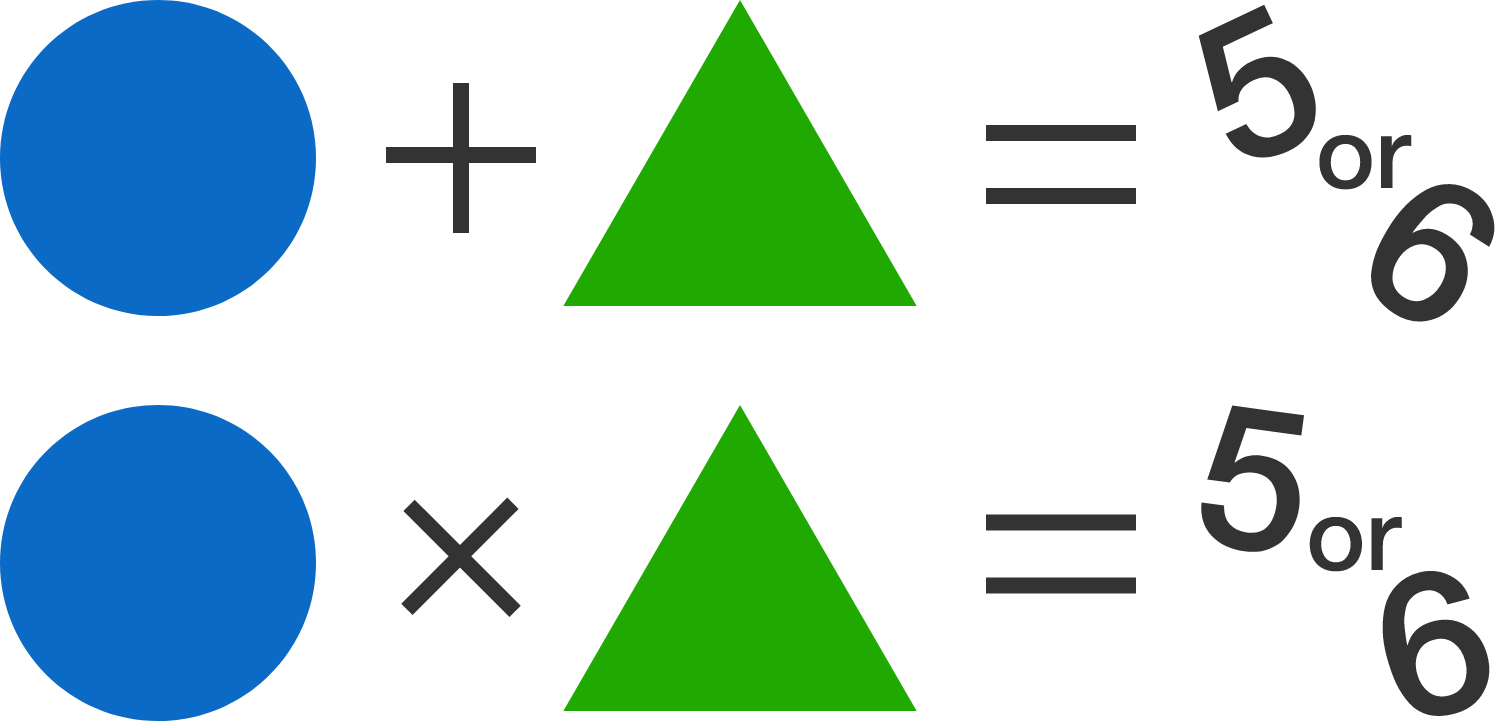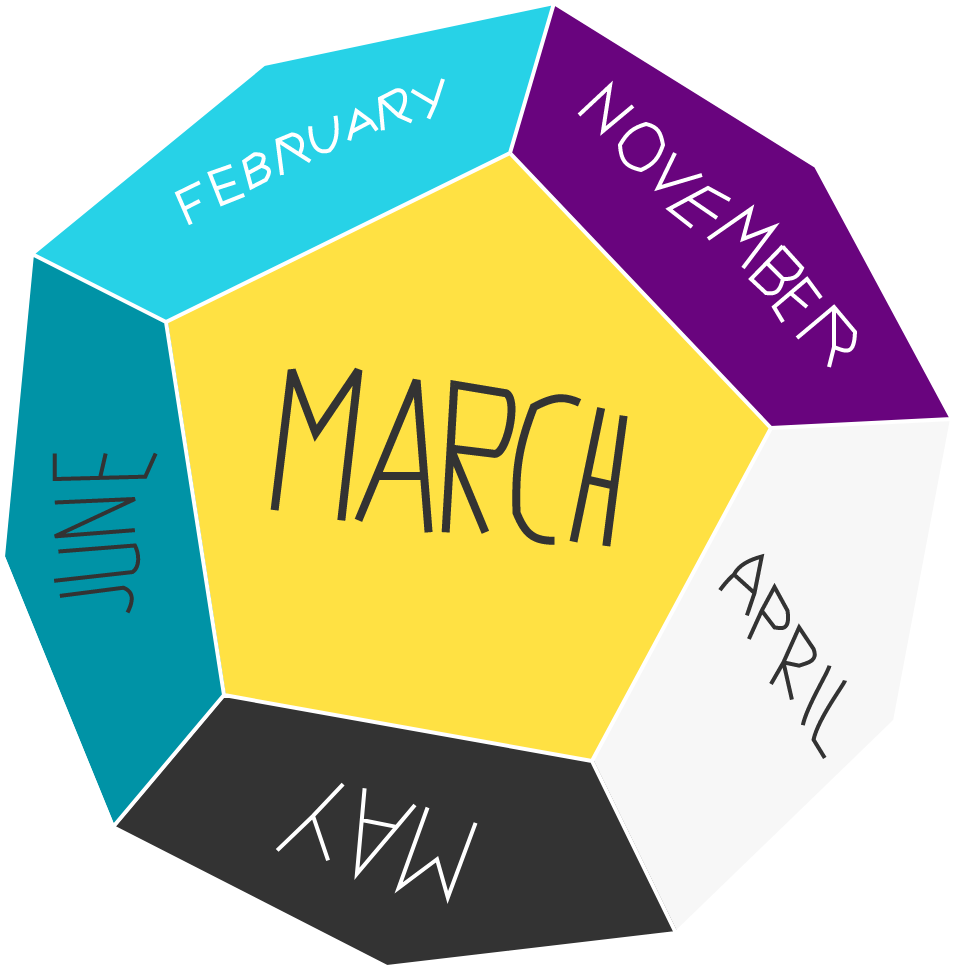# Problems of the Week

Contribute a problem

# 2017-03-06 Basic

Playing with the numbers in today's date (March 2017), I started to write out this equation:

$\frac{ {\color{#3D99F6}3}} { {\color{#20A900}20}} - \frac{ {\color{#3D99F6}3}}{ {\color{#D61F06}17}} = - \frac{{\color{#3D99F6}3}}{{\color{#20A900}20}} \times \frac{{\color{#3D99F6}3}}{{\color{#D61F06}17}}$

Is the equation true or false?A chain made of identical links is hanging between two points (indicated by the hooks). We can increase the length of the chain by adding more links to it until the chain becomes very heavy and breaks.

At which point is the chain most likely to break?

Find the last 3 digits of $\large 123456789\times 987654321.$

Hint: We do not need to multiply the 9 digit integers.The sum of the 2 numbers is either 5 or 6.
The product of the 2 numbers is either 5 or 6 as well.

Which of the following cannot be a value for either of the 2 numbers?A dodecahedron has 12 pentagonal faces, and companies have used them to create dodecahedral calendars.

Is it possible to place the months of the dodecahedral calendar such that consecutive months are placed on adjacent faces?

Note: This would allow us to roll the calendar model along an edge from one month to the next. The connection between adjacent months begins with Jan-Feb and ends with Dec-Jan.

×